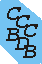Computational Chemistry Comparison and Benchmark DataBase Release 22 (May 2022) Standard Reference Database 101 National Institute of Standards and Technology Home All data for one species Geometry Experimental Calculated Comparisons Bad Calculations Tutorials and Explanations Vibrations Experimental Calculated Scale factors Reactions Entropies Ions List Ions Energy Electron Affinity Proton Affinity Ionization changes point group Experimental One molecule all properties One property a few molecules Geometry Vibrations Energy Electrostatics Reference Data Calculated Energy Optimized Reaction Internal Rotation Orbital Nuclear repulsion energy Correlation Ion Excited State Basis Set Extrapolation Geometry Vibrations Frequencies Zero point energy (ZPE) Scale Factors Bad Calculations Electrostatics Charges Dipole Quadrupole Polarizability Spin Entropy and Heat Capacity Reaction Lookup by property Comparisons Geometry Vibrations Energy Entropy Electrostatics Ion Resources Info on Results Calculations Done Basis functions used I/O files Glossary Conversion Forms Links NIST Links External links Thermochemistry Tutorials Vibrations Entropy Energy Electrostatics Geometry Cost Bad Calculations FAQ Help Units Choose Units Explanations Credits Just show me Summary Using List Recent molecules Molecules Geometry Vibrations Energy Similar molecules Ions, Dipoles, etc. Index of CCCBDB Feedback You are here: Resources > Tutorials > Electrostatics > Quadrupoles

# Tutorial on Electric Quadrupole Moments

## What is it?

Any distribution of charges can be described using a multipole expansion. The multipole expansion breaks the distribution down into the total charge, the dipole, the quadrupole, the octapole, etc.

### Formulas

(I'm still working on this page) see for example: http://en.wikipedia.org/wiki/Electric_Quadrupole

## Practical considerations

Molecular electric quadrupole moments in general are derived (sometimes it seems in rather involved ways) from some other measured property. They are derived from the measurement of the second virial coefficient of gases (a measure of how non-ideal a gas is) and spectroscopic observations changes in the rotational energy levels when the molecule is in a magnetic or electric field. In both cases the quadrupole moment is a fudge factor that tweaks some other property. There is a physical basis for the expansion used in both cases, but I would not expect the values obtained from two such different measurements, with different random and systematic errors, to agree within the stated uncertainties of each measurement.

## Primitive? Traceless?

Some of the confusion over factors of two, and "primitive" versus "traceless" comes from this use of the quadrupole moment as a coefficient in different expansions describing different properties. The electric quadrupole moment can be described as a 3x3 symmetric matrix with components:
 Qxx Qxy Qxz Qxy Qyy Qyz Qxz Qyz Qzz
This has 6 different values. However, the traceless version is used to omit the spherical component. An experimental measurement will observe the part of the quadrupole that is different from spherical. Just to make it a little more confusing instead of just subtracting the trace the diagonal elements of the "traceless" matrix are defined as:
xx' = (3*xx-trace)/2
yy' = (3*yy-trace)/2
zz' = (3*zz-trace)/2
I believe this comes from the use of the components in particular equations.

One reference that discusses how the "gas-property people" use the quadrupole is in: "Theory of Molecular Fluids Volume1: Fundamentals" C.G. Gray and K.E. Gubbins, Oxford University Press, New York, 1984

## Relation between cartesian and spherical components

monopole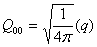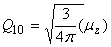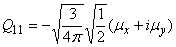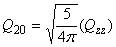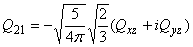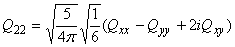However one often wants just the magnitude of the quadrupole, where the magnitude of a general multipole moment is defined as: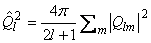The previous equations are from "Theory of Molecular Fluids Volume1: Fundamentals" C.G. Gray and K.E. Gubbins, Oxford University Press, New York, 1984